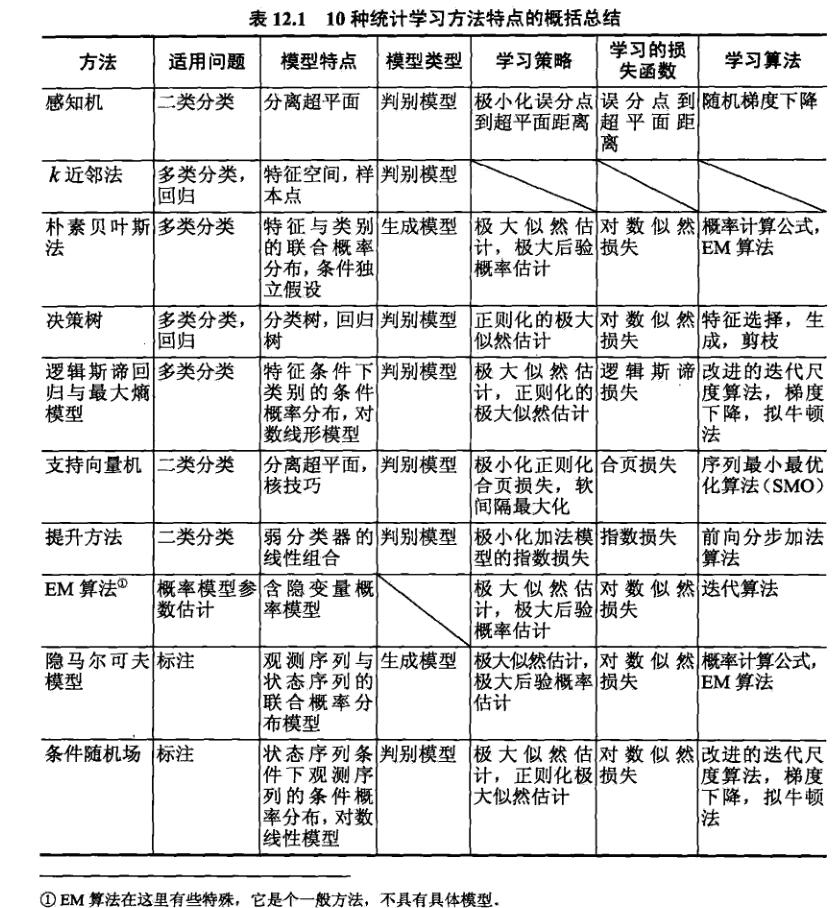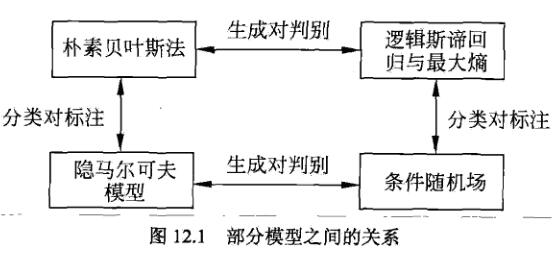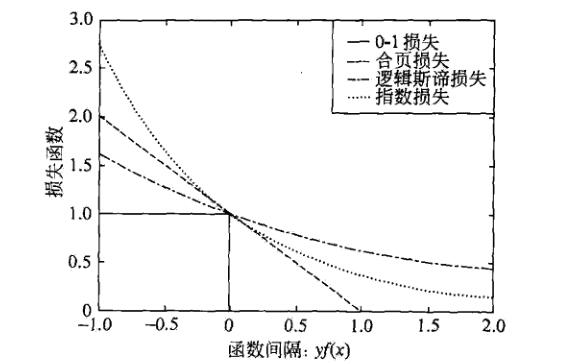# 李航-统计学习方法-笔记-12：总结

## 10种统计学习方法特点的概括总结## 适用问题

EM算法是含有隐变量的概率模型的一般学习算法，可用于生成模型的非监督学习。

## 模型## 学习策略

$[1-yf(x)]_+$

$\log [1+exp(-yf(x))]$

$exp(-yf(x))$$\min_{f \in H} \frac{1}{N} \sum_{i=1}^N L(y_i, f(x_i)) + \lambda J(f)$

SVM用$$L_2$$范数表示模型复杂度。

$- \log P(y \ | \ x)$

## 学习算法

SVM学习，可解凸二次规划的对偶问题，有SMO等方法。

boosting利用学习的模型是加法模型，损失函数是指数函数的特点，启发式地从前向后逐步学习模型，以达到逼近优化目标函数的目的。

EM算法是一种迭代的求解含因变量概率模型参数的方法，它的收敛性可以保证，但不能保证收敛到全局最优。

SVM，逻辑斯谛回归与最大熵模型，CRF是凸优化问题，全局最优解保证存在。而其它学习问题则不是凸优化问题。

posted @ 2019-06-05 15:58  PilgrimHui  阅读(1297)  评论(0编辑  收藏  举报